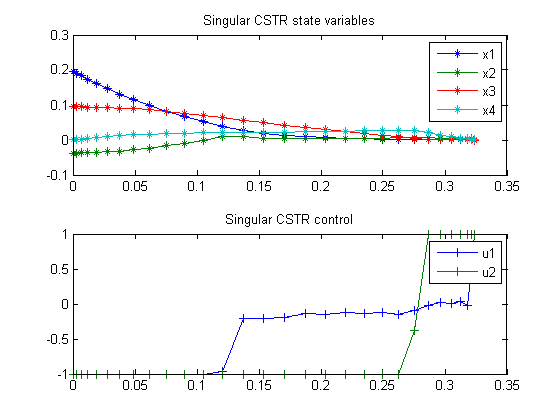100  Singular CSTR

ITERATIVE DYNAMIC PROGRAMMING, REIN LUUS

10.4 Nonlinear two-stage CSTR problem

CHAPMAN & HALL/CRC Monographs and Surveys in Pure and Applied Mathematics

100.1  Problem Formulation

Find u over t in [0; t_F ] to minimize:

 J = x(tF)′*x(tF) + tF

(the state variables are moved to bounds)

subject to:

 dx1 dt
= −3*x1+g1
 dx2 dt
= −11.1558*x2+g1−8.1558*(x2+0.1592)*u1
 dx3 dt
= 1.5*(0.5*x1x3)+g2
 dx4 dt
= 0.75*x2−4.9385*x4+g2−3.4385*(x4+0.122)*u2

g1 = 1.5e7*(0.5251−x1)*exp(−
 10 x2+0.6932
)−
1.5e10*(0.4748+x1)*exp(−
 15 x2+0.6932
) − 1.4280
g2 = 1.5e7*(0.4236−x2)*exp(−
 10 x4+0.6560
)−
1.5e10*(0.5764+x3)*exp(−
 15 x4+0.6560
) − 0.5086

The initial condition are:

 x(0) = [0.1962  −0.0372  0.0946  0]
 −1 <= u(1:2) <= 1

Reference: 

100.2  Problem setup

toms t t_f
p = tomPhase('p', t, 0, t_f, 30);
setPhase(p)

tomStates x1 x2 x3 x4
tomControls u1 u2

% Initial guess
x0 = {t_f == 0.3
icollocate({x1 == 0.1962; x2 == -0.0372
x3 == 0.0946; x4 == 0})
collocate({u1 == 0; u2 == 0})};

% Box constraints
cbox = {0.1 <= t_f <= 100
-1 <= collocate(u1) <= 1
-1 <= collocate(u2) <= 1};

% Boundary constraints
cbnd = {initial({x1 == 0.1962; x2 == -0.0372
x3 == 0.0946; x4 == 0})
final({x1 == 0; x2 == 0
x3 == 0; x4 == 0})};

% ODEs and path constraints
g1 = 1.5e7*(0.5251-x1).*exp(-10./(x2+0.6932)) ...
- 1.5e10*(0.4748+x1).*exp(-15./(x2+0.6932)) - 1.4280;
g2 = 1.5e7*(0.4236-x2).*exp(-10./(x4+0.6560)) ...
- 1.5e10*(0.5764+x3).*exp(-15./(x4+0.6560)) - 0.5086;

ceq = collocate({
dot(x1) == -3*x1+g1
dot(x2) == -11.1558*x2+g1-8.1558*(x2+0.1592).*u1
dot(x3) == 1.5*(0.5*x1-x3)+g2
dot(x4) == 0.75*x2-4.9385*x4+g2-3.4385*(x4+0.122).*u2});

% Objective
objective = t_f;

100.3  Solve the problem

options = struct;
options.name = 'Singular CSTR';
solution = ezsolve(objective, {cbox, cbnd, ceq}, x0, options);
t  = subs(collocate(t),solution);
x1 = subs(collocate(x1),solution);
x2 = subs(collocate(x2),solution);
x3 = subs(collocate(x3),solution);
x4 = subs(collocate(x4),solution);
u1 = subs(collocate(u1),solution);
u2 = subs(collocate(u2),solution);
Problem type appears to be: lpcon
Starting numeric solver
===== * * * =================================================================== * * *
TOMLAB - Tomlab Optimization Inc. Development license  999001. Valid to 2011-02-05
=====================================================================================
Problem: ---  1: Singular CSTR                  f_k       0.324402684069356410
sum(|constr|)      0.000000010809098017
f(x_k) + sum(|constr|)      0.324402694878454410
f(x_0)      0.299999999999999990

Solver: snopt.  EXIT=0.  INFORM=1.
SNOPT 7.2-5 NLP code
Optimality conditions satisfied

FuncEv    1 ConstrEv   75 ConJacEv   75 Iter   42 MinorIter  427
CPU time: 0.562500 sec. Elapsed time: 0.594000 sec.

100.4  Plot result

subplot(2,1,1)
plot(t,x1,'*-',t,x2,'*-',t,x3,'*-',t,x4,'*-');
legend('x1','x2','x3','x4');
title('Singular CSTR state variables');

subplot(2,1,2)
plot(t,u1,'+-',t,u2,'+-');
legend('u1','u2');
title('Singular CSTR control');